# Numerical Analysis in a Lid-Driven Square Cavity with Hemispherical Obstacle in the Bottom

Numerical Analysis in a Lid-Driven Square Cavity with Hemispherical Obstacle in the Bottom

Abbas Fadhil Khalaf Farhan Lafta Rashid*Ali Basem Mohammed H. Abbas

Petroleum Engineering Department, College of Engineering, University of Kerbala, Karbala 56001, Iraq

Air Conditioning Engineering Department, Faculty of Engineering, Warith Al-Anbiyaa University, Karbala 56001, Iraq

Medical Physics Department, Al-Mustaqbal University College, Babylon 51001, Iraq

Corresponding Author Email:
farhan.lefta@uokerbala.edu.iq
Page:
1639-1647
|
DOI:
https://doi.org/10.18280/mmep.090625
25 September 2022
|
Accepted:
20 December 2022
|
Published:
31 December 2022
| Citation

OPEN ACCESS

Abstract:

Incompressible fluid flow research uses lid-driven cavity as a benchmark issue to measure computer simulation accuracy. Flow within a hollow includes recirculation, turbulence, eddies, instability, impingement, flow separation, attachment with walls (moving and stationary), fluid entrapment in the recirculation area, and other fluid flow phenomena. In this article, forced convection in a lid-driven square cavity with a sliding top wall and hemispherical obstruction is mathematically illustrated. This chamber filled with Newtonian fluid was exposed to a moving wall (5, 10, 15, and 20 m/s), as selected in the literature. The finite volume technique using the SIMPLER algorithm is used to solve the complete governing equations with the Boussinesq approximation, whereas the analytical approach uses the parallel flow assumption. The moving wall disrupts the cavity's flow field, according to the results. Also, moving wall produces great mixing between the flow field below it and the hollow. The static pressure fluctuates from 0.6 m to 1 m (contact with the moving wall). Also, dynamic pressure increases linearly until 0.7 m, then decreases linearly until 1 (contact with the moving wall). In addition, the inner surface's velocity varies randomly along the location while the others remain constant.

Keywords:

lid-driven cavity, square cavity, separation, attachment with walls, moving or stationary walls

1. Introduction

Lid-driven cavity flow is a vital field of research in fluid mechanics because it often occurs in engineering problems like short-dwell and flexible blade coaters. This kind of flow configuration may be utilized as a benchmark problem for many numerical approaches because of its simple geometry and complicated flow behaviours. This issue and its effects on engineering have received considerable attention in recent years in a number of pertinent studies. Surprisingly, fluid flow with solid particles is important in many industrial applications, including grain drying, particle separation, fluidized beds, the manufacturing of milk powder, and coal combustion [1-3]. The lid-driven flow in a cubical cavity has always received a lot of attention because to the wide variety of applications they have, including crystal formation, electronic card cooling, multi-screen structures used in nuclear reactors, and food processing. Understanding the flow structure inside cubical cavities caused by a cylindrical shape in the center is thought to be one of the fundamental challenges of fluid dynamics because the two- and three-dimensional cavity flows are canonical problems of fluid dynamics qualitatively presenting some of the key features responsible for transition to periodic then turbulence in a wide variety of confined flow situations [4, 5]. As a result, the scientific community has shown enormous interest in the deep exploration of physical insights as well as the flow behaviour in lid-driven enclosures. Additionally, different-shaped enclosures (circle, square, triangle, etc.) may be employed to regulate or split the fluid flow . The lid driven cavity has been the focus of several investigations in the literature because of its natural allure as well as its ability to assess the accuracy and efficacy of newly created numerical methods. Two-dimensional lid driven cavities were the subject of the first numerical research . Ghia et al.  implemented a stream-function vorticity technique and a connected implicit multigrid method also established exact calculations in such settings. Rectangular cavities with varied aspect ratios were also explored [9, 10], even though the investigations mentioned above were restricted to square cavities with unity aspect ratios. Lattice Boltzmann technique was used by Bhopalam et al.  to calculate flows in double-sided cross shaped lid-driven cavities. First, using a complex geometry called the double-sided cross-shaped lid-driven cavity with antiparallel uniform wall motion, a symmetrized variant of the staggered lid-driven cavity, is examined. The modification of Reynolds numbers and oscillation frequencies for uniform and oscillating wall movements have effectively captured the motion and production of main and secondary vortices. Samantaray et al.  used a large eddy simulation (LES) in conjunction with a dynamic Smagorinsky model (DSM) to simulate the flow within a cubic lid-driven cavity at a Reynolds number of 10,000 and elicit the turbulence that characterizes that flow. The joint probability distribution function and quadrant analysis both demonstrate that there are more coherent structures close to the downstream wall and that the turbulence is anisotropic. Hu  used the lattice Boltzmann technique to examine the motion of a neutrally buoyant elliptical particle in a lid-driven square cavity. The limit cycle, which is produced by the elliptical particle's inertia, the confinement of the square cavity's borders, and vortex behavior, is the most evident feature of the motion of the elliptical particle in the square cavity. The limit cycle is forced toward the center of the square cavity as the particle size increases due to increased boundary confinement. Using a dynamic Smagorinsky model, Samantaray and Das  performed a large eddy simulation of incompressible flow within a cubic lid driven cavity over a range of Reynolds numbers (Re=1000, 3200, 5000, 10000, and 15000). The nature of turbulence is discovered to be non-homogeneous and anisotropic even at low (Re=3200), and non-homogeneity grows as Re rises. This is known as an anisotropic invariant map (AIM). The core portion of the cavity's turbulence moves toward isotropy as Re increases. To ascertain the effect of material parameters, obstacle geometry, and adhesion force on the deposition profiles, Roy et al.  conducted a number of simulations and parameterization investigations. The majority of the time, particles are caught in biofilm and areas around cavities with low Okubo-Weiss numbers, where the relative vorticity dominates over the local strains. By using an Eulerian-Lagrangian approach, Golkarfard et al.  studied numerically the deposition of aerosol particles in laminar mixed-convection flow in a lid-driven hollow with two heated barriers. 2000 particles that were originally distributed randomly in the flow regime and were tracked using lagrangian particle transport calculations were likewise considered to have no impact on the fluid. Based on the numerical findings, free convection was a significant factor in the deposition. As a key outcome, it is shown that deposition declines as the Richardson number rises.

Two main objectives of the current inquiry are to provide a benchmark for a problem involving a lid-driven square cavity with a top moving wall and a hemispherical impediment at the bottom and to elucidate the issue mechanics that underlie the investigation. To the best of the authors' knowledge, this problem is the most prevalent one that they have found in the relevant literature. These two goals are meant to be effectively accomplished.

2. Problem Description and Mathematical Formulation

The top (lid) surface of the two-dimensional square cavity, which has a width of 1 m, is used to mimic fluid flow. It flows consistently from left to right at speeds of 5, 10, 15, and 20 m/s (as taken in previous works), shown in Figure 1 and Table 1. The cavity's bottom and two vertical walls are insulated, and the lid surface is kept at a constant 25℃ temperature. On the bottom wall, there is a hemispherical obstruction that is kept at a constant temperature of 25℃. This hemispherical obstruction has a radius of 0.25 meters. The border was defined according to the criteria listed below [17-20]:

(1) The axis of symmetry, also known as axial symmetry.

(2) The rotating periodicity, often known as the sides of the wedge.

(3) Inlet: $u=(U, 0,0), \nabla p . n=0, \tau=0$

(4) Outlet: $\nabla \mathrm{u}_{\mathrm{i}} \cdot \mathrm{n}=0, \mathrm{p}=0, \nabla \tau_{\mathrm{ij}} \cdot \mathrm{n}=0$

The conservations of mass, momentum, and energy are the governing equations for incompressible, steady, and two-dimensional flow, and they may be expressed as follows [21-38]:

$\frac{\partial u}{\partial x}+\frac{\partial v}{\partial y}=0$         (1)

$u \frac{\partial u}{\partial x}+v \frac{\partial u}{\partial y}=-\frac{1}{\rho} \frac{\partial P}{\partial x}+\frac{\mu}{\rho}\left(\frac{\partial^2 u}{\partial x^2}+\frac{\partial^2 u}{\partial y^2}\right)$          (2)

$u \frac{\partial v}{\partial x}+v \frac{\partial v}{\partial y}=-\frac{1}{\rho} \frac{\partial P}{\partial y}+\frac{\mu}{\rho}\left(\frac{\partial^2 v}{\partial x^2}+\frac{\partial^2 v}{\partial y^2}\right)+g \beta\left(T-T_C\right)$       (3)

$\frac{\partial\left(\rho u C_P T\right)}{\partial x}+\frac{\partial\left(\rho v C_P T\right)}{\partial y}=k\left(\frac{\partial^2 T}{\partial x^2}+\frac{\partial^2 T}{\partial y^2}\right)$          (4)

If the x- and y-components of velocity are u and v, g is the gravitational acceleration vector, $\rho$ is the fluid density, P is the pressure, $\mu$ is the fluid dynamic viscosity, $\ \beta$ is the coefficient of thermal expansion (β=1/Tf), T is the fluid temperature, Tf is the reference fluid temperature, and k is the fluid thermal conductivity. With the exception of the Boussinesq approximation, the fluid characteristics remain constant.

## 1.png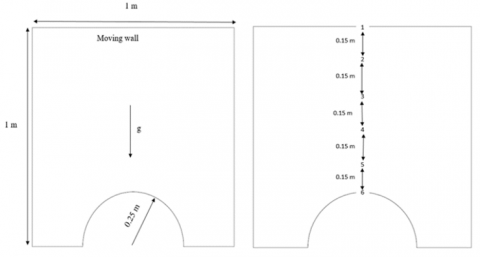Figure 1. Dimensions of the case study

Table 1. Coordinates of the case study

 x y z Point no. 0.5 1 0.0066 1 0.5 0.85 0.0066 2 0.5 0.7 0.0066 3 0.5 0.55 0.0066 4 0.5 0.44 0.0066 5 0.5 0.25 0.0066 6
3. Simulation Procedure and the Numerical Test

Before the numerical simulation of the current study can be carried out, there is a checklist of tasks that need to be finished and validated. These two major components are what you should focus on while attempting to summarize these points:

(1) Creating the grids and analysing the density of the individual components of the grids are two procedures that may be taken to cut down on the amount of error that is present in the numerical results.

(2) Determining whether or not the numerical model that was used provides accurate results.

Gambit is the name of the code that is used to generate and build the grids that make up the area that is being analysed. The structure of the grid in its final form is seen in Figure 2 after having been finished. The value of the ratio was used in each case in order to arrive at a conclusion about the density of the components. Table 2 presents the results of this investigation for your perusal. It is feasible to reach the conclusion that the quantity of components in case 2 is enough to provide the required degree of fulfilment. Grid independence test is the term that has been given to this specific step.

Table 2. Grid independency test for the top moving wall velocity of 5 m/s

 Component density Case Total cell number Velocity (m/s) Difference % 0.1 1 43075 4.87 0.24 2 100550 4.90 0.09 3 223300 4.98 0.08 4 321500 5.00 0.08 0.2 1 42020 4.76 0.48 2 86240 4.82 0.025 3 174660 4.87 0.023 4 271100 4.99 0.023 0.3 1 33176 4.91 2.30 2 70552 4.93 0.22 3 140315 4.94 0.22 4 221000 4.92 0.22

## 2.png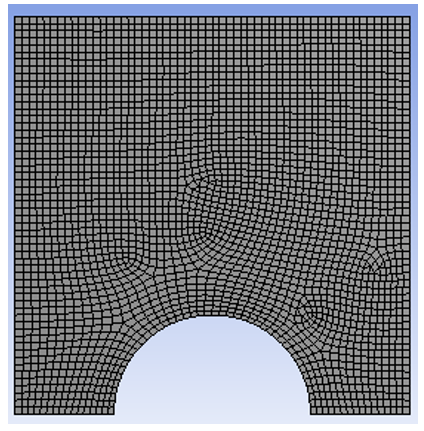Figure 2. Mesh generation

## 3.png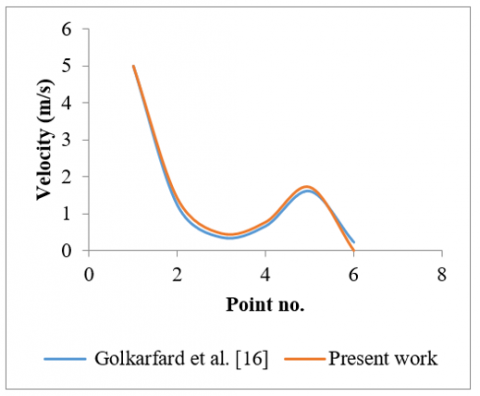Figure 3. Comparison of velocity distribution between current study results and Golkarfard al.  for top moving wall velocity of 5 m/s

The numerical code ANSYS-Fluent was used to address the issue raised by this inquiry. Using the finite volume method, the code integrates the starting conditions (eqs. 1 and 2) before translating the differential equations. The convective terms of the matrix system are solved using the high-resolution method. The SIMPLEC method was used to relate the velocity and pressure. The findings of the computation may be used when the inaccuracy is equal to (10-6) for energy equations and (10-8) for momentum equations. The numerical method presented in this research is used to solve the lid-driven square cavity with hemispherical obstacle and a top moving wall velocity of 5 m/s developed by Golkarfard et al. . As can be seen from the contrast in Figure 3, there is a very high level of trust. These comparisons confirm the correctness of the method.

4. Results and Discussion

4.1 Velocity and pressure distribution

Figure 4 shows the impact of a moving wall on the contours of the pressure and velocity distribution in the square hollow. In this illustration, it was believed that the top wall would move at a speed of 5 m/s. It is evident that the flow field within the hollow is clearly disturbed by the shifting of the wall. Additionally, it can be inferred from these data that a moving wall provides great mixing between the cavity and the flow field underneath it. Physically, there are two mechanisms that cause forced convection: The first is when air enters a hollow. The second consequence, however, results from the additional shear stress brought on by the hollow wall's displacement. Additionally, it is clear from the flow field that the streamlines are significantly impacted by the wall movement's direction. They move and gather close to the moving wall as a result. The strong flow circulation near the moving wall is caused by the shear force that is created as a result of the wall's movement. The stagnant area at the lower corners of the cavity's side wall is eliminated as a result of the wall's movement. This is another advantage of the movement for a wall, because it promotes the development of vortices within the cavity. The similar pattern can be seen in Figures 5, 6, and 7 for the top moving wall with speeds of 10, 15, and 20 m/s, respectively.

The pressure on a wall's surface that is static or moving uniformly in one direction Dynamic pressure is the force exerted when a surface moves in the same direction as a fluid-moving wall. As a result, Figure 8 depicts the static pressure distribution along the cavity's vertical-mid axis. This graphic demonstrates the variation in static pressure, with the least value occurring at 0.6 meters and the largest value occurring at 1 meters (contact with the moving wall). Figures 9, 10, and 11 with top moving wall velocities of 10, 15, and 20 m/s, respectively, show the same trend. The dynamic pressure distribution along the cavity's vertical-mid axis is seen in Figure 5-8. From this graph, it can be observed that dynamic pressure increases linearly until it reaches its maximum value at 0.7 m, after which it decreases linearly to point 1 (contact with the moving wall). Figures 12, 13, and 14 for top moving wall velocities of 10, 15, and 20 m/s, respectively, show the similar trend.

The moving bottom wall, left wall, right wall, interior surface, and inner surface along the location are all shown in Figure 15 with their respective velocities. The internal surface's velocity, as can be seen, fluctuates arbitrarily along the location but remains constant for the others. Similar trends may be seen in Figures 16, 17, and 18, with top moving wall velocities of 10, 15, and 20 m/s, respectively.

The static pressure for the moving wall, bottom wall, left wall, right wall, and interior surface is shown in Figure 19. The static pressure for the moving wall can be seen to oscillate along its location, starting at zero at the bottom of a cavity, becoming vacuum at 0.6 m, and then reaching its maximum at the top (the place at which it moves). Because of how the top moving wall affected the air next to the right wall and caused it to depart the cavity, the right wall at point 1 has a static pressure that starts at vacuum and increases to 11 Pa. The interior surfaces have random static pressure along the positions. Figures 20, 21, and 22 with top moving wall velocities of 10, 15, and 20 m/s, respectively, show the same result.

The dynamic pressure distribution for the moving wall, bottom wall, left wall, right wall, and inner surface is shown in Figure 23. The moving wall has a minimal value at the bottom of the cavity, reaches its maximum at 0.7 m (9 Pa), and then decreases to 7 Pa at the top of the zone, as can be observed. Because of how the top moving wall affected the air next to the right wall and caused it to flee the cavity, the right wall at point 1 had a dynamic pressure that started at zero and increased to 5.8 Pa. Along the internal surface, dynamic pressure is distributed randomly. The similar pattern can be seen in Figures 24, 25, 26, and 27 which depict top moving wall velo cities of 10, 15, and 20 m/s, respectively.

## 4.png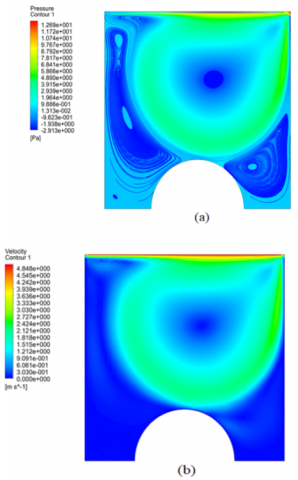Figure 4. Contours of (a) Pressure distribution; (b) Velocity distribution, for moving wall velocity of 5 m/s

## 5.pngFigure 5. Contours of (a) Pressure distribution; (b) Velocity distribution, for moving wall velocity of 10 m/s

## 6.png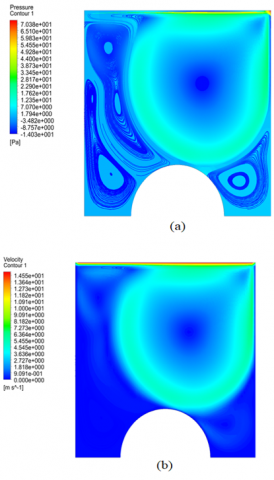Figure 6. Contours of (a) Pressure distribution (b) Velocity distribution, for moving wall velocity of 15 m/s

## 7.png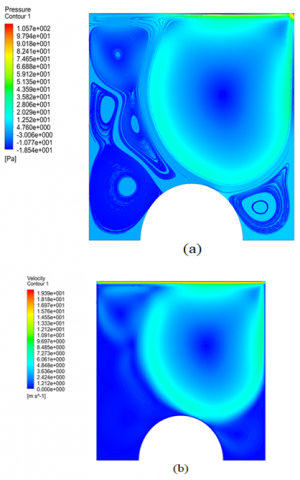Figure 7. Contours of (a) Pressure distribution (b) Velocity distribution, for moving wall velocity of 20 m/s

## 8.png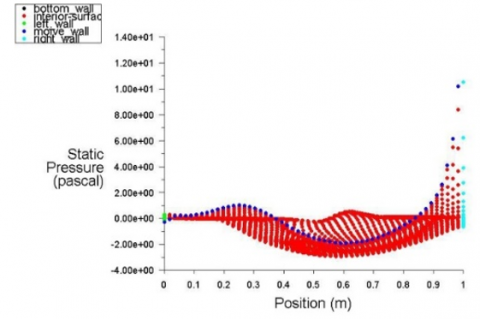Figure 8. Static pressure distribution along the position for moving wall velocity of 5 m/s

## 9.png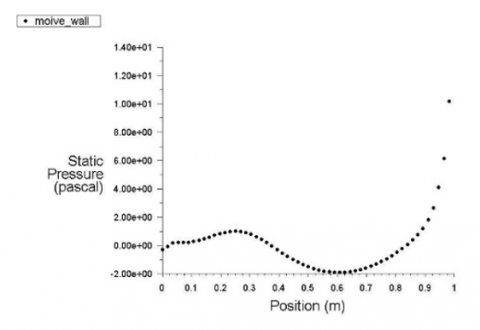Figure 9. Static pressure distribution along the position for moving wall velocity of 10 m/s

## 10.png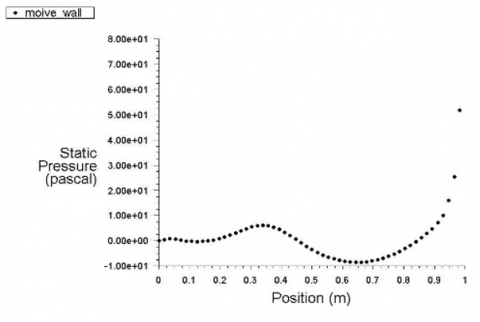Figure 10. Static pressure distribution along the position for moving wall velocity of 15 m/s

## 11.png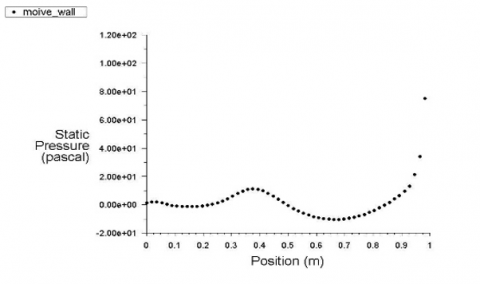Figure 11. Static pressure distribution along the position for moving wall velocity of 20 m/s

## 12.png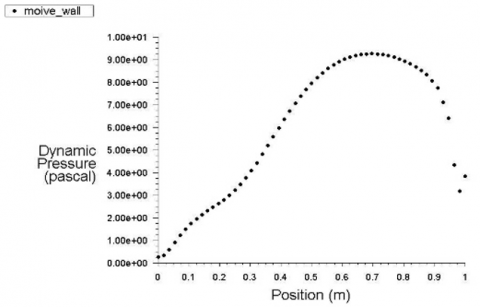Figure 12. Dynamic pressure distribution along the position for moving wall velocity of 10 m/s

## 13.png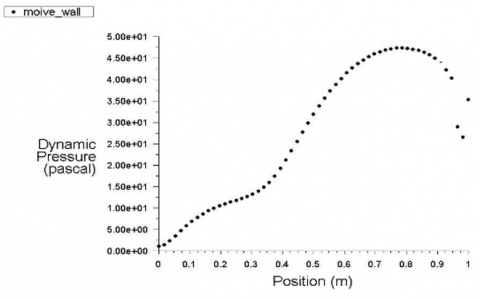Figure 13. Dynamic pressure distribution along the position for moving wall velocity of 15 m/s

## 14.png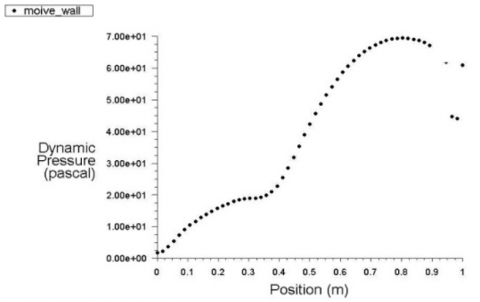Figure 14. Dynamic pressure distribution along the position for moving wall velocity of 20 m/s

## 15.png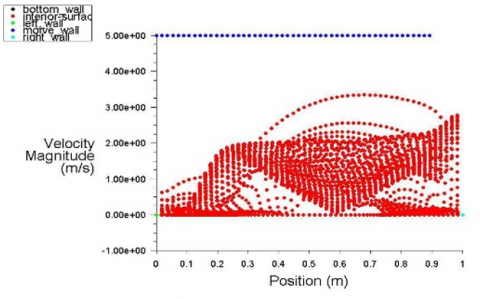Figure 15. Velocity magnitude along the position for moving wall velocity of 5 m/s

## 16.pngFigure 16. Velocity magnitude along the position for moving wall velocity of 10 m/s

## 17.png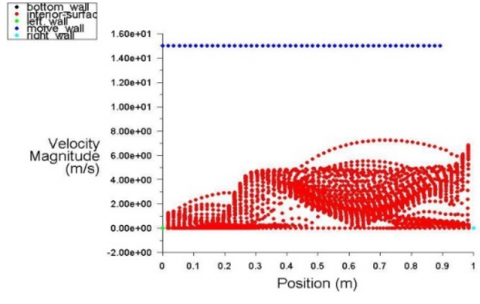Figure 17. Velocity magnitude along the position for moving wall velocity of 15 m/s

## 18.png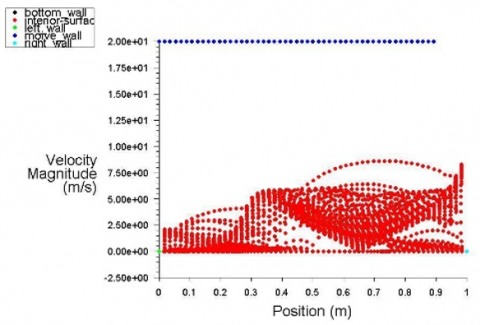Figure 18. Velocity magnitude along the position for moving wall velocity of 20 m/s

## 19.png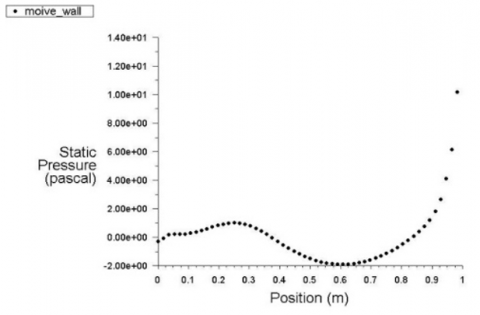Figure 19. Static pressure distribution along the position for moving wall velocity of 5 m/s

## 20.png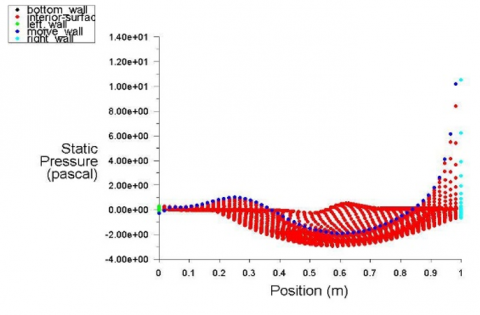Figure 20. Static pressure distribution along the position for moving wall velocity of 10 m/s

## 21.png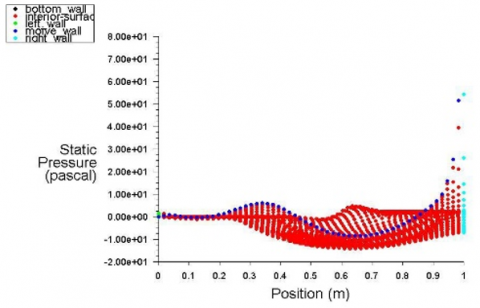Figure 21. Static pressure distribution along the position for moving wall velocity of 15 m/s

## 22.png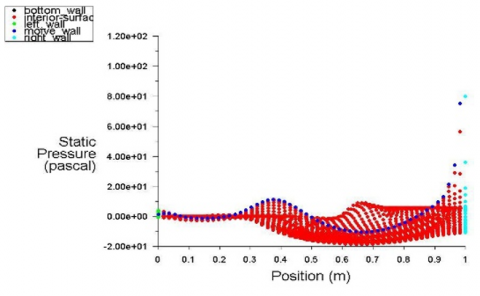Figure 22. Static pressure distribution along the position for moving wall velocity of 20 m/s

## 23.png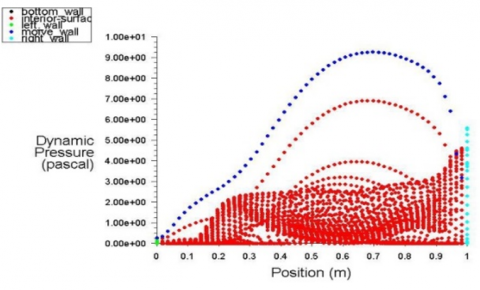Figure 23. Dynamic pressure distribution along the position for moving wall velocity of 5 m/s

## 24.pngFigure 24. Dynamic pressure distribution along the position for moving wall velocity of 10 m/s

## 25.png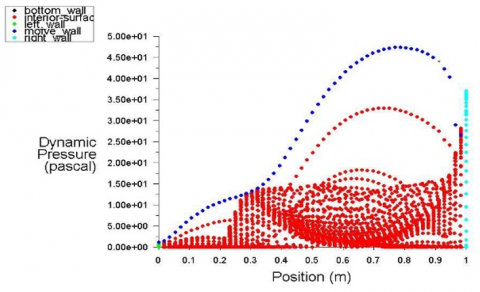Figure 25. Dynamic pressure distribution along the position for moving wall velocity of 15 m/s

## 26.png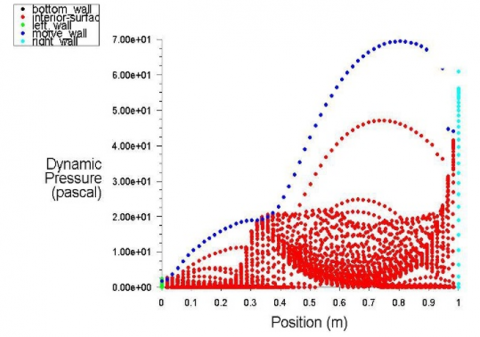Figure 26. Dynamic pressure distribution along the position for moving wall velocity of 20 m/s

4.2 Comparison for varying moving wall velocity

Comparisons for varying the velocity and pressure distribution of the top moving wall are conceivable. The contours of velocity and pressure distribution are shown in Figures 27 and 28, respectively, for top moving wall velocities of 5, 10, 15, and 20 m/s.

Figure 29 displays the distribution of air velocity along the location (point number). It indicates that the velocity should be spread symmetrically throughout the two sides of the cavity, with point 1 (contact with moving wall) having the greatest value and points 6 and 7 having the lowest (at the top of the hemispherical obstacle). These results demonstrate that an increase in moving wall speed improves air flow speed.

Figure 30 displays the pressure distribution along the location (point number). This shows that the pressure should be spread symmetrically over the cavity's two sides, with points 1 and 6 having the greatest values and 3 and 4 having the lowest values. Despite being equal to that at point 1, the pressure at position 6 has the opposite sign. These results demonstrate that the pressure distribution grows as the velocity of the moving wall increases.

## 27.png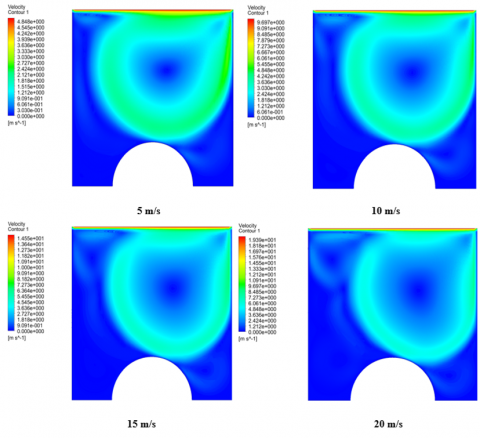Figure 27. Contours of velocity distribution for different moving wall velocity

## 28.png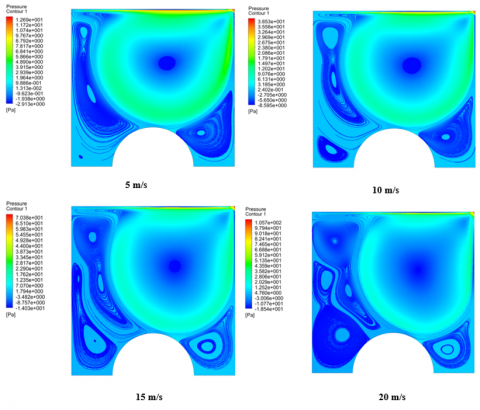Figure 28. Contours of pressure distribution for different moving wall velocity

## 29.pngFigure 29. Air flow velocity distribution along the position for different moving wall velocity

## 30.pngFigure 30. Pressure distribution along the position for different moving wall velocity

5. Conclusions

Lid-driven cavity flow is an important topic of research in fluid mechanics because it often occurs in engineering problems like short-dwell and flexible blade coaters. This kind of flow arrangement may serve as a benchmark problem for many numerical algorithms because of its simple geometry and complicated flow behaviours. A classic benchmark issue, fluid flow in a lid-driven cavity exhibits a range of flow characteristics, including corner eddies, bifurcation, and transition to turbulence, while having a basic geometric structure. The following conclusions might be drawn from this work:

1. The flow field within the hollow is clearly disturbed by the moving wall. Additionally, it may be inferred that the moving wall creates a mixing between the cavity and the flow field underneath it.

2. The static pressure varies, with a minimum value of 0.6 meters and a maximum value of 1 meters (contact with the moving wall).

3. While the other speeds are consistent along the location, the inside surface's speed varies at random.

4. The moving wall fluctuates along its location, starting at zero at the bottom of a hollow, becoming vacuum at 0.6 m, and reaching its maximum at the top (the place at which it moves).

5. The velocity should be evenly distributed throughout both sides of the cavity, with point 1 (contact with moving wall) having the greatest value and points 6 having the lowest (at the top of the hemispherical obstacle).

6. The two sides of the cavity should experience an equal distribution of pressure, with the highest value occurring at points 1 and 6 and the lowest at points 3 and 4.

References

 Kosinski, P., Kosinska, A., Hoffmann, A.C. (2009). Simulation of solid particles behaviour in a driven cavity flow. Powder Technol., 191: 327-339. https://doi.org/10.1016/j.powtec.2008.10.025

 Sidik, N.A., Attarzadeh, M.R. (2012). The use of cubic interpolation method for transient hydrodynamics of solid particles. International Journal of Engineering Science, 51: 90-103. https://doi.org/10.1016/j.ijengsci.2011.08.014

 Safdari, A., Kim, K.C. (2014). Lattice Boltzmann simulation of solid particles behavior in a three-dimensional lid-driven cavity flow. Computers & Mathematics with Applications, 68(5): 606-621. https://doi.org/10.1016/j.camwa.2014.07.004

 Souayeh, B., Cheikh, N., Beya, B. (2016). Numerical simulation of three-dimensional natural convection in a cubic enclosure induced by an isothermally-heated circular cylinder at different inclinations. Int. J. of Thermal Sci., 110: 325-339. https://doi.org/10.1016/j.ijthermalsci.2016.08.003

 Ravnik, J., Škerget, L. (2015). A numerical study of nanofluid natural convection in a cubic enclosure with a circular and an ellipsoidal cylinder. Int. J. Heat Mass Transf., 89: 596-605. https://doi.org/10.1016/j.ijheatmasstransfer.2015.05.089

 Hammami, F., Souayeh, B., Cheikh, N., Beya, B. (2017). Computational analysis of fluid flow due to a two-sided lid driven cavity with a circular cylinder. Computers & Fluids, 156: 317-328. https://doi.org/10.1016/j.compfluid.2017.07.017

 Burggraf, O.R. (1966). Analytical and numerical studies of the structure of steady separated flows. Journal of Fluid Mechanics, 24(1): 113-151. https://doi.org/10.1017/S0022112066000545

 Ghia, U., Ghia, K.N., Shin, C.T. (1982). High-Re solutions for incompressible flow using the Navier-Stokes equations and a multigrid method. Journal of Computational Physics, 48(3): 387-411. https://doi.org/10.1016/0021-9991(82)90058-4

 Cortes, A.B., Miller, J.D. (1994). Numerical experiments with the lid driven cavity flow problem. Computers and Fluids, 23: 1005-1027. https://doi.org/10.1016/0045-7930(94)90002-7

 Pan, F., Acrivos, A. (1967). Steady flows in rectangular cavities. Journal of Fluid Mechanics, 28(4): 643-655. https://doi.org/10.1017/S002211206700237X

 Bhopalam, R.S., Perumal, D.A., Yadav, A.K. (2018). Computation of fluid flow in double sided cross-shaped lid-driven cavities using Lattice Boltzmann method. European Journal of Mechanics - B/Fluids, 70: 46-72. https://doi.org/10.1016/j.euromechflu.2018.01.006

 Samantaray, D., Das, M.K., Patel, D.K. (2020). Turbulence characteristics of high Reynolds number flow inside a three-dimensional cubic lid-driven cavity. European Journal of Mechanics - B/Fluids, 84: 23-39. https://doi.org/10.1016/j.euromechflu.2020.05.009

 Hu, J. (2021). Motion of a neutrally buoyant elliptical particle in a lid-driven square cavity. European Journal of Mechanics-B/Fluids, 85: 124-133. https://doi.org/10.1016/j.euromechflu.2020.09.008

 Samantaray, D., Das, M.K. (2019). Nature of turbulence inside a cubical lid-driven cavity: Effect of Reynolds number. International Journal of Heat and Fluid Flow, 80: 108498. https://doi.org/10.1016/j.ijheatfluidflow.2019.108498

 Roy, N., Wijaya, K.P., Götz, Th., Sundar, S. (2021). A mathematical model governing the short-range transport of microplastic particles in a lid-driven cavity with an obstacle. Communications in Nonlinear Science and Numerical Simulation, 101: 105893. https://doi.org/10.1016/j.cnsns.2021.105893

 Golkarfard, V., Nasab, S.A., Ansari, A.B., Bagheri, G.H. (2012). Numerical investigation on deposition of solid particles in a lid-driven square cavity with inner heated obstacles. Advanced Powder Technology, 23(6): 736-743. https://doi.org/10.1016/j.apt.2011.09.006

 Rashid, F.L., Hussein, A.K., Malekshah, E.H., Abderrahmane, A., Guedri, K., Younis, O. (2022). Review of heat transfer analysis in different cavity geometries with and without nanofluids. Nanomaterials, 12: 2481. https://doi.org/10.3390/nano12142481

 Rashid, F.L., Fakhrulddin, S.K., Eleiwi, M.A., Hussein, A.K., Tahseen, T.A., Younis, O., Ahmed, M.I. (2022). CFD simulation in thermal-hydraulic analysis of air flow on different attack angles of row flat tube. Frontiers in Heat and Mass Transfer, 19(6). https://doi.org/10.5098/hmt.19.6

 Redha, Z.A., Rashid, F.L. (2021). Heat transfer enhancement in subchannel geometry of pressurized water reactor using water-based Yttrium Oxide Nanofluid. International Journal of Heat and Technology, 39(3): 979-986. https://doi.org/10.18280/ijht.390335

 Rashid, F.L., Redha, Z.A., Mohammed, A.A. (2021). Thermal analysis on the fuel rod assemblies with triangular and square array using new nanofluid. Journal of Engineering Science and Technology, 16(5): 3801-3821.

 Rashid, F.L., Talib, S.M., Hussein, A.K., Younis, O. (2022). An experimental investigation of double pipe heat exchanger performance and exergy analysis using air bubble injection technique. Jordan Journal of Mechanical and Industrial Engineering, 16(2): 195-204.

 Hussein, E.Q., Sadeq, B.R., Rashid, F.L. (2020). Fluid structure interaction of non–return valve using CFD technique. Journal of Mechanical Engineering Research and Developments, 43(7): 137-148.

 Al-Jibory, M.W., Rashid, F.L., Hussein, H.Q. (2020). Review of heat transfer enhancement in air-cooled turbine blades. International Journal of Scientific & Technology Research, 9(4): 3123-3130.

 Al-Jibory, M.W., Rashid, F.L., Talib, S.M. (2020). Review on cooling enhancement of different shape gas turbine ribbed blade with thermal barrier coating. International Journal of Scientific Research and Engineering Development, 3(1): 313-329.

 Altaie, A., Hasan, M.R., Rashid, F.L. (2014). Numerical heat transfer and turbulent flow in a circular tube fitted with opened rings having square cross section. Journal of Basic and Applied Scientific Research, 4(11): 28-36.

 Rashid, F.L., Altaie, A., Hasan, M.R. (2014). Numerical investigation of heat transfer enhancement in a circular tube using ribs of separated ports assembly. European Scientific Journal.

 Altaie, A., Hasan, M.R., Rashid, F.L. (2015). Numerical investigation of heat transfer enhancement in a circular tube with rectangular opened rings. Bulletin of Electrical Engineering and Informatics, 4(1): 18-25. https://doi.org/10.11591/eei.v4i1.331

 Altaie, A., Hasan, M., Rashid, F.L. (2015). Heat transfer enhancement in a circular tube using ribs with middle arm. Elixir International Journal.

 Altaie, A., Hasan, M., Rashid, F.L. (2015). Numerical investigation in a circular tube to enhance turbulent heat transfer using opened rings-triangular cross section. Journal of Babylon University/ Engineering Sciences, 23(3): 798-807.

 Al-Jibory, M.W., Rashid, F.L., Hussein, H.Q. (2018). Heat transfer augmentation in gas turbine blade rectangular passages using circular ribs with fins. Journal of University of Babylon, Engineering Sciences, 26(1): 247-258.

 Al-Jibory, M.W., Rashid, F.L., Talib, S.M. (2018). Numerical investigation of heat transfer enhancement in ribbed elliptical passage. Journal of Engineering and Applied Sciences, 13(17): 7223-7234. https://doi.org/10.3923/jeasci.2018.7223.7234

 Aljibory, M.W., Rashid, F.L, Alais, S.M. (2018). An experimental and numerical investigation of heat transfer enhancement using annular ribs in a tube. IOP Conference Series: Materials Science and Engineering, 433(1): 012057. https://doi.org/10.1088/1757-899X/433/1/012057

 Rashid, F.L., Al-Jibory, M.W., Talib, S.M. (2018). Numerical investigation of heat transfer augmentation in elliptical passage with different rib geometries and aspect ratios. International Journal of Mechanical Engineering and Technology, 9(13): 1390-1409.

 Al-Jibory, M.W., Rashid, F.L., Talib, S.M. (2018). An experimental investigation of heat transfer enhancement in elliptical passage fitted with different rib geometries. International Journal of Mechanical Engineering and Technology, 9(13): 1033-1048.

 Hussein, H.Q., Al-Jibory, M.W., Rashid, F.L. (2020). Heat transfer enhancement of gas turbine blades using coated ribs with nanocomposite materials. Journal of Mechanical Engineering Research and Developments, 43(6): 9-22.

 Rashid, F.L., Fakhrulddin, S.K., Eleiwi, M.A., Hussein, A.K., Tahseen, T.A., Younis, O., Ahmed, M.I. (2022). CFD simulation in thermal-hydraulic analysis of air flow on different attack angles of row flat tube. Frontiers in Heat and Mass Transfer, 19(6): 1-8. https://doi.org/10.5098/hmt.19.6

 Abbas, A.S., Mohammed, A.A. (2022). Augmentation of plate-fin heat exchanger performance with support of various types of fin configurations. Mathematical Modelling of Engineering Problems, 9(5): 1406-1414. https://doi.org/10.18280/mmep.090532

 Nancy, M., Stephen, E.A. (2022). Modelling and analysis of the cone coupling problem using optimization. Mathematical Modelling of Engineering Problems, 9(5): 1385-1392. https://doi.org/10.18280/mmep.090529